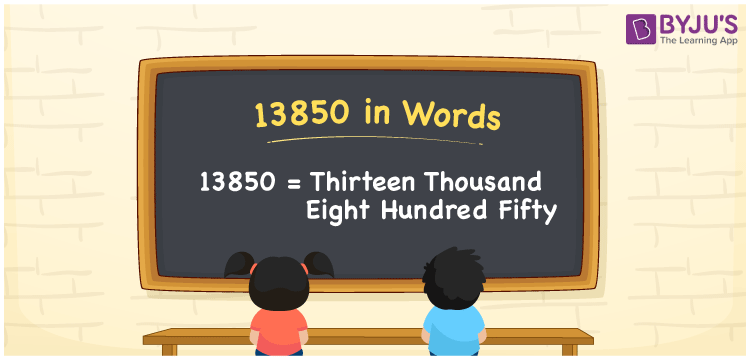# 13850 in Words

We can write 13850 in words as Thirteen thousand eight hundred fifty. If you paid a bill of Rs. 13850 in a shop, you can say, “I spent Thirteen thousand eight hundred fifty rupees at the shop”. Thus, we can express 13850 as Thirteen thousand eight hundred fifty. In this article, you will learn how to write the number 13850 in words with the help of a place value chart.

 13850 in words Thirteen thousand eight hundred fifty Thirteen thousand eight hundred fifty in numerical form 13850

## 13850 in English Words

Generally, we use the English alphabet to express numbers in words. Thus, we can express 13850 in English words as “Thirteen thousand eight hundred fifty”.## How to Write 13850 in Words?

The number 13850 has five digits. So, to convert 13850 into words, we need to create a place value chart with five columns as given below.

 Ten thousands Thousands Hundreds Tens Ones 1 3 8 5 0

Here, ones = 0, tens = 5, hundreds = 8, thousands = 3, ten thousand = 1

These digits can be expanded as follows.

1 × Ten thousand + 3 × Thousand + 8 × Hundred + 5 × Ten + 0 × One

= 1 × 10000 + 3 × 1000 + 8 × 100 + 5 × 10 + 0 × 1

= 10000 + 3000 + 800 + 50

= Ten Thousand + Three thousand + Eight hundred + Fifty

= Thirteen thousand + Eight hundred fifty {since ten + three = thirteen}

= Thirteen thousand eight hundred fifty

13850 is a natural number that is the successor of 13849 and predecessor of 13851.

13850 in words – Thirteen thousand eight hundred fifty

Is 13850 an odd number? – No

Is 13850 an even number? – Yes

Is 13850 a prime number? – No

Is 13850 a composite number? – Yes

Is 13850 a perfect square number? – No

Is 13850 a perfect cube number? – No

## Frequently Asked Questions on 13850 in Words

Q1

### How do you write 13850 in words?

We can write 13850 in English words as Thirteen thousand eight hundred fifty.
Q2

### How to write Rs. 13850 in words on a cheque?

On a cheque, we can write the amount Rs. 13850 in words as “Thirteen thousand eight hundred fifty rupees only”.
Q3

### Find 13850 – 5850. Write the answer in words.

13850 – 5850 = 8000 Hence, the value of 13850 – 5850, i.e., 8000 in words is Eight thousand.
Test your Knowledge on 13850 in Words